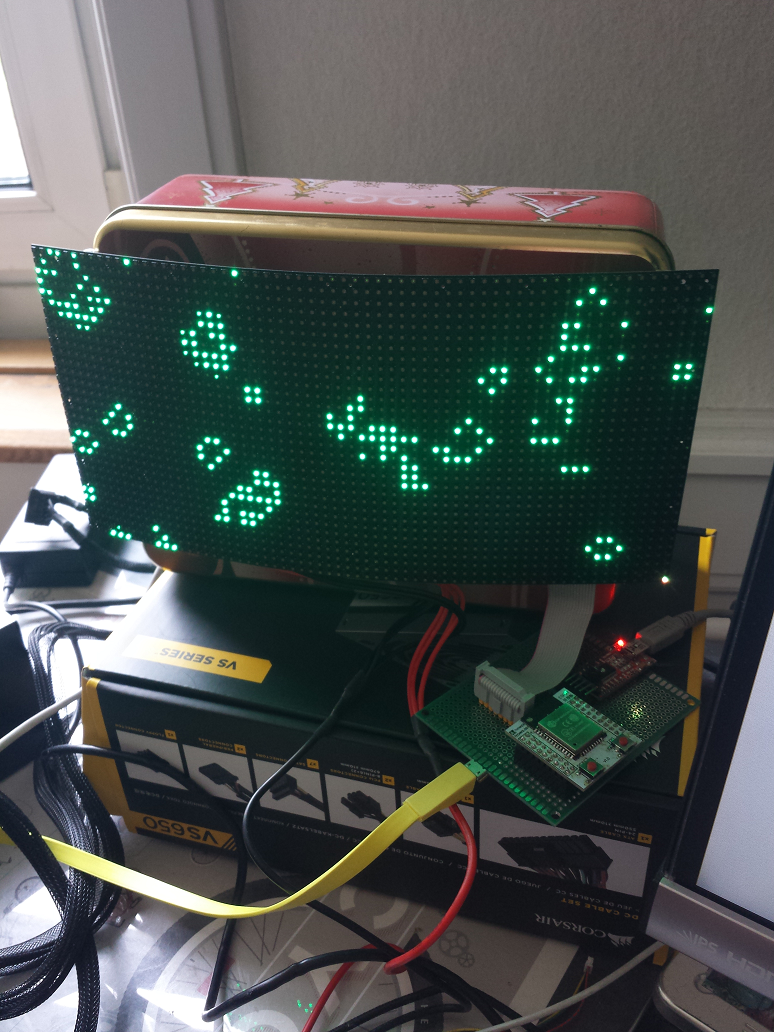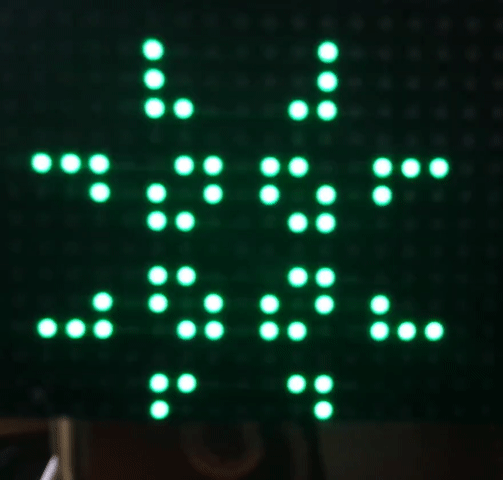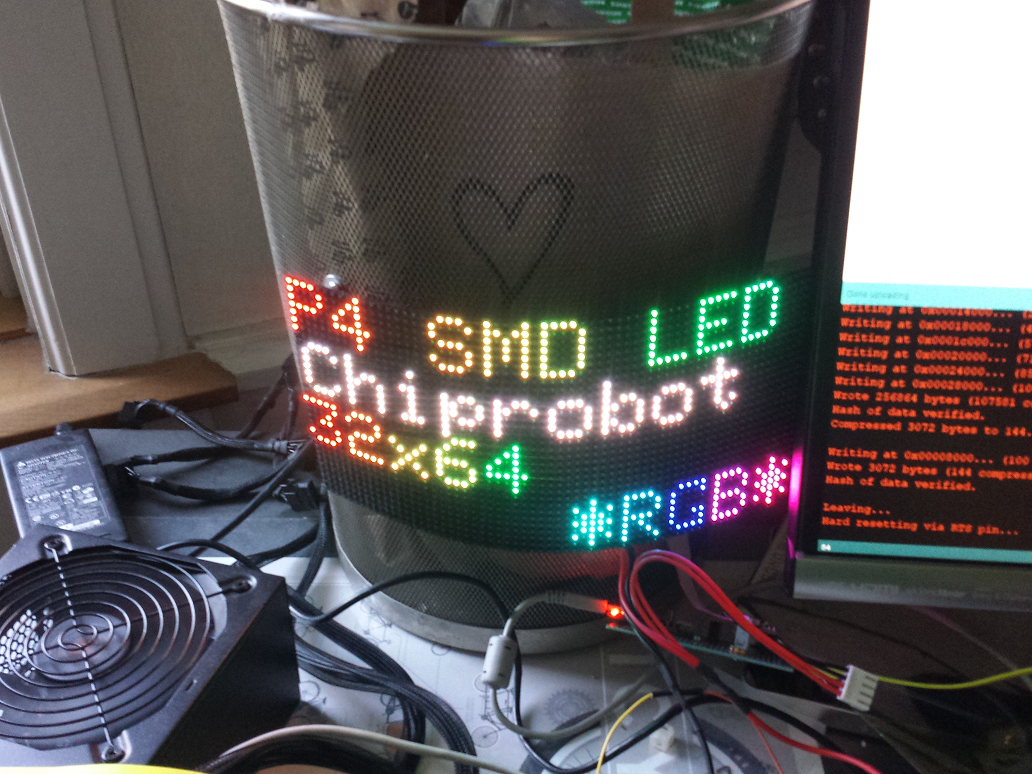# EPS32 "Life is Life" (Conways Life)

Here is a test of a flexible P4 RGB LED 64x32 matrix screen.My trusty "Conway's Life" program was used as the test culprit along with a variant of the

adafruits P3 matrix driver Library  (arduino/mega/etcetc)

ESP32 P3/P4 matrix driver Library

Its blisteringly fast on the ESP32 , the gif below has been slowed down BTW.

Its one of the recuring loops that can happen , " Repetition is the Mother of Skill"ESP32  "Life is Life" Conways Life /*  * Conway's "Life", adapted for ESP32  */ #include    // Core graphics library #include // constructor with default pin wiring P3RGB64x32MatrixPanel matrix;   byte world; long density = 20; int polltime; void setup() {  matrix.begin();  randomSeed(analogRead(0));  randomscreen();  } void loop() {  if (millis()-polltime >=50)  { displayoutput(); polltime=millis();};   } int neighbours(int x, int y) {  return          world[(x + 1)        % 64][  y                   ]         +          world[ x                          ][ (y + 1)        % 32]  +          world[(x + 64 - 1) % 64][  y                   ]        +          world[ x                          ][ (y + 32 - 1) % 32]  +          world[(x + 1)        % 64][ (y + 1)        % 32]  +          world[(x + 64 - 1) % 64][ (y + 1)        % 32]  +          world[(x + 64 - 1) % 64][ (y + 32 - 1) % 32]  +          world[(x + 1) % 64       ][ (y + 32 - 1) % 32] ; } void randomscreen() {     for (int y = 0; y < 32; y++) {     for (int x = 0; x < 64; x++) {       if (random(100) < density) {world[x][y] = 1; }       else                       {world[x][y] = 0;}       world[x][x] = 0;     }   }  } void displayoutput(){   for (int y = 0; y < 32; y++) {     for (int x = 0; x < 64; x++) {       // Default is for cell to stay the same       world[x][y] = world[x][y];       int count = neighbours(x, y);       if (count == 3 && world[x][y] == 0)               { world[x][y] = 1;matrix.drawPixel(x, y, matrix.color444(0,255,0)); } //new cell       if ((count < 2 || count > 3) && world[x][y] == 1) { world[x][y] = 0;matrix.drawPixel(x, y, matrix.color444(0,0,0)); } //cell dies     }   }   // swap next generation into place   for (int y = 0; y < 32; y++) {     for (int x = 0; x < 64; x++) {       world[x][y] = world[x][y];     }    }   }

...and those interested in the colours.... its wrapped around my metal bin (it comes with magnetic feet stand-offs)## Comment viewing options